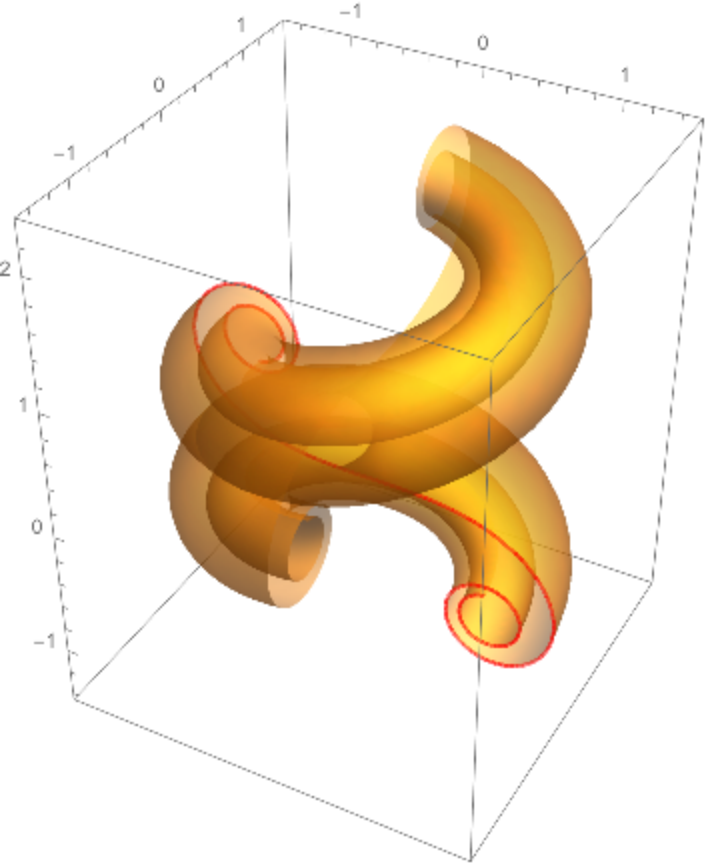#Function Repository Resource:

# CurvatureTorsionPlot3D

Plot a curve defined by its curvature and torsion

Contributed by: Alfred Gray
 ResourceFunction["CurvatureTorsionPlot3D"][{κ,τ},{t,tmin,tmax},{a0,p,q,r}] plots the curve c defined by its curvature κ and torsion τ, written as functions of t and having initial conditions c(a0)=p,c'(a0)=q and c''(a0)=κ(a0)r. ResourceFunction["CurvatureTorsionPlot3D"][{κ,τ},{t,tmin,tmax}] plots the curve using default initial conditions. ResourceFunction["CurvatureTorsionPlot3D"][{{κ1,τ1},…},{t,tmin,tmax}] plots several curves defined by their curvatures κi and torsions τi.

## Details and Options

Default initial conditions for the curve c are c(a0)={0,0,0}, c'(a0)={1,0,0} and c''(a0)=κ(a){0,1,0}, where κ is the curvature.
ResourceFunction["CurvatureTorsionPlot3D"] has the same options as ParametricPlot and NDSolve.

## Examples

### Basic Examples

Zero torsion gives a plane curve:

 In:=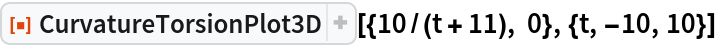Out=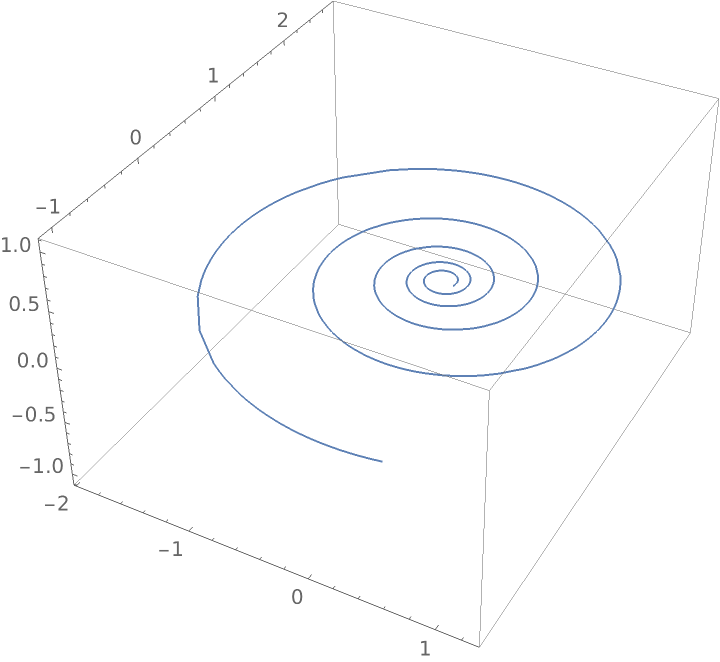Constant curvature and torsion gives a helix:

 In:=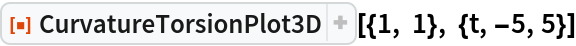Out=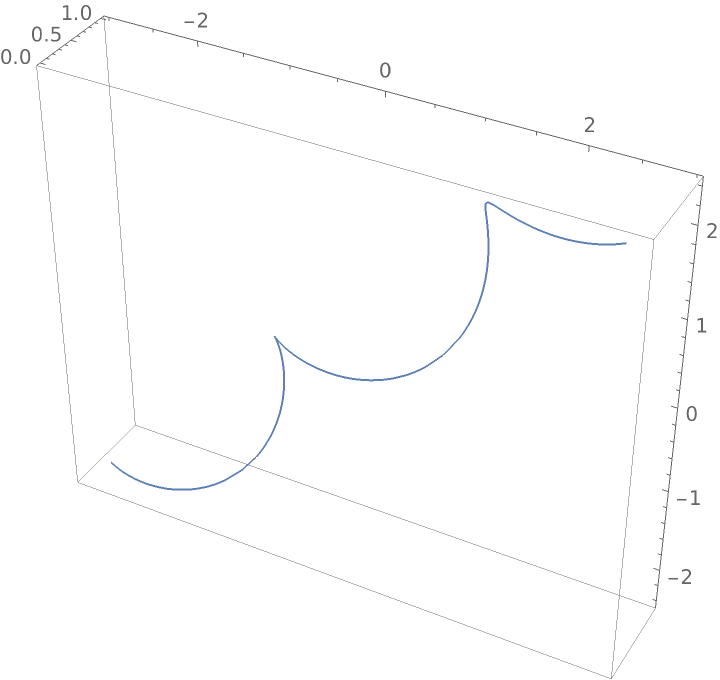Linear curvature and constant torsion:

 In:=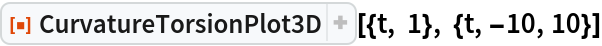Out=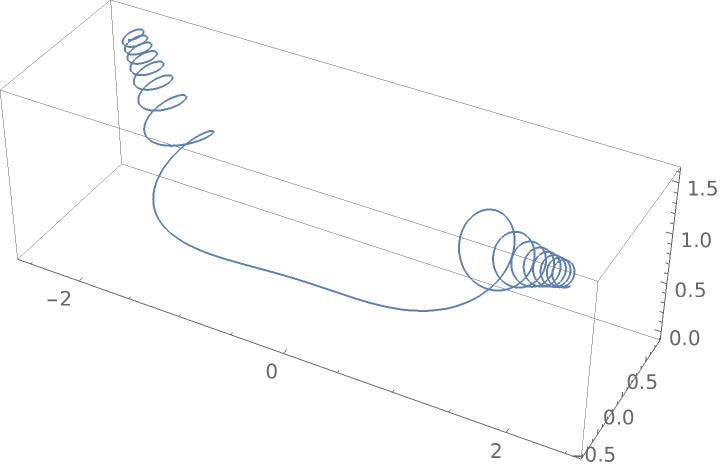Linear curvature and torsion:

 In:=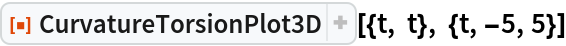Out=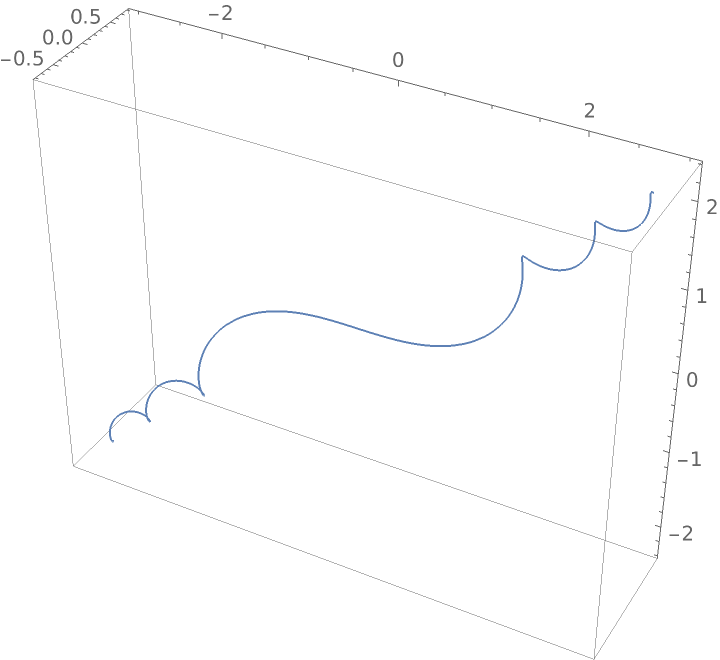Constant curvature and linear torsion:

 In:=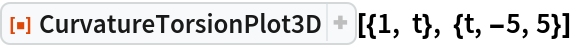Out=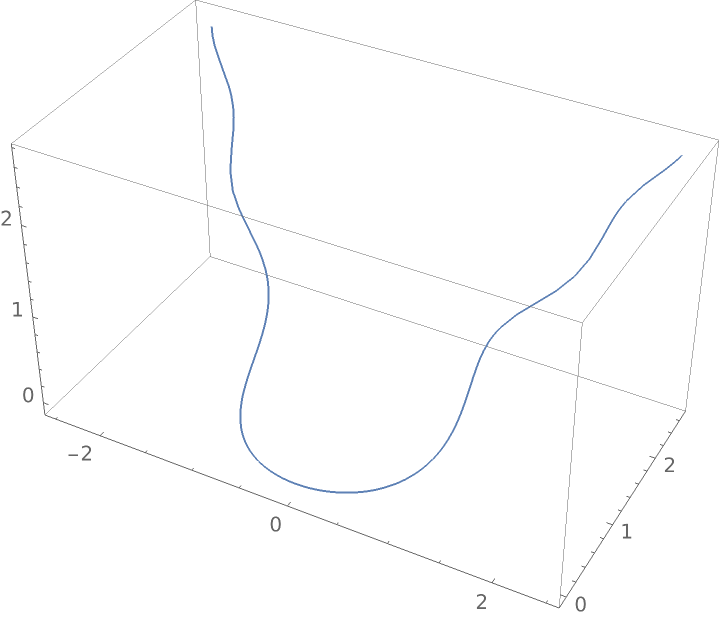Constant curvature and sinusoidal torsion:

 In:=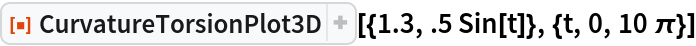Out=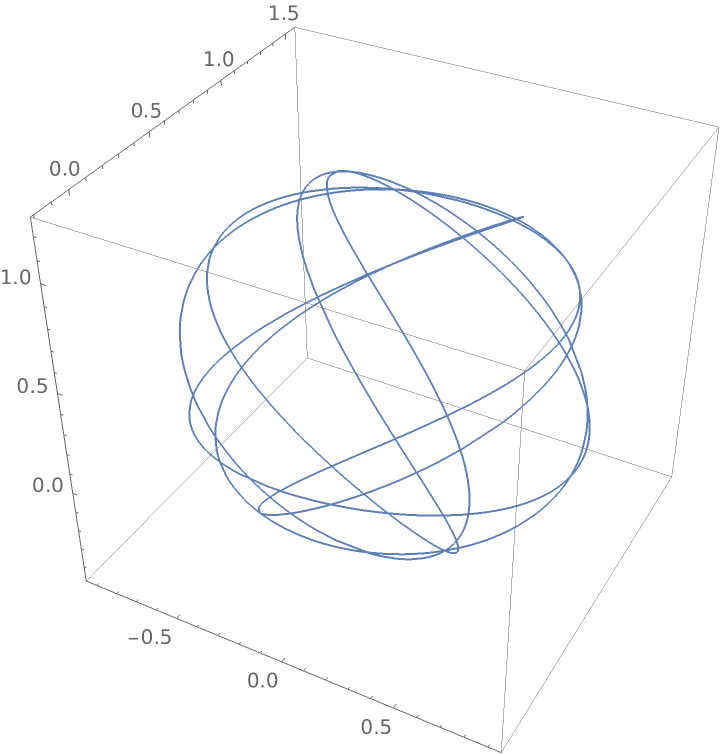Sine–cosine curvature and torsion:

 In:=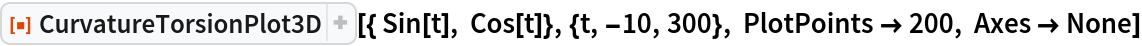Out=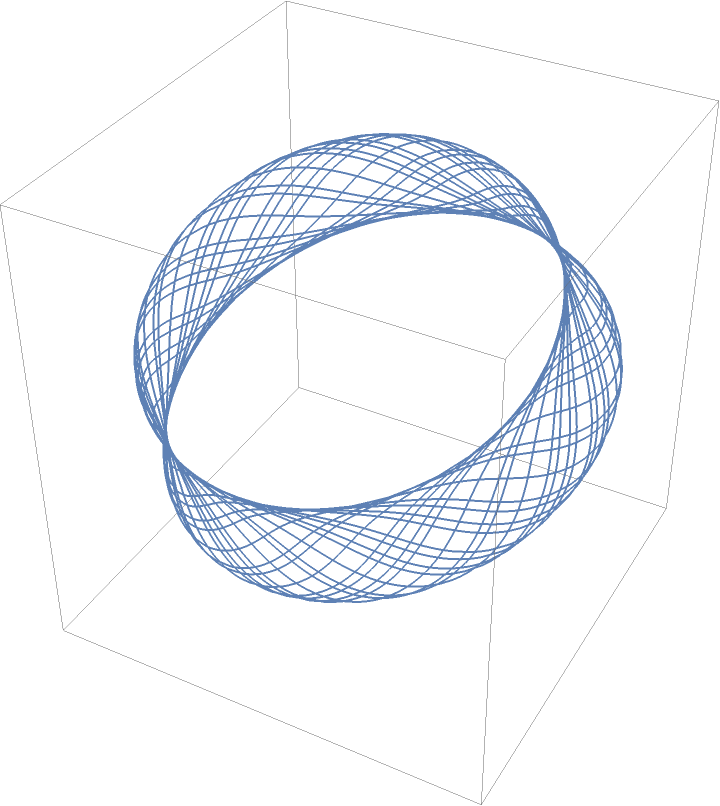Using a sawtooth wave curve:

 In:=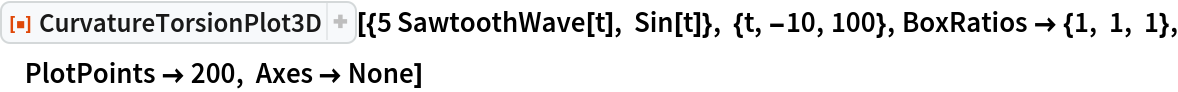Out=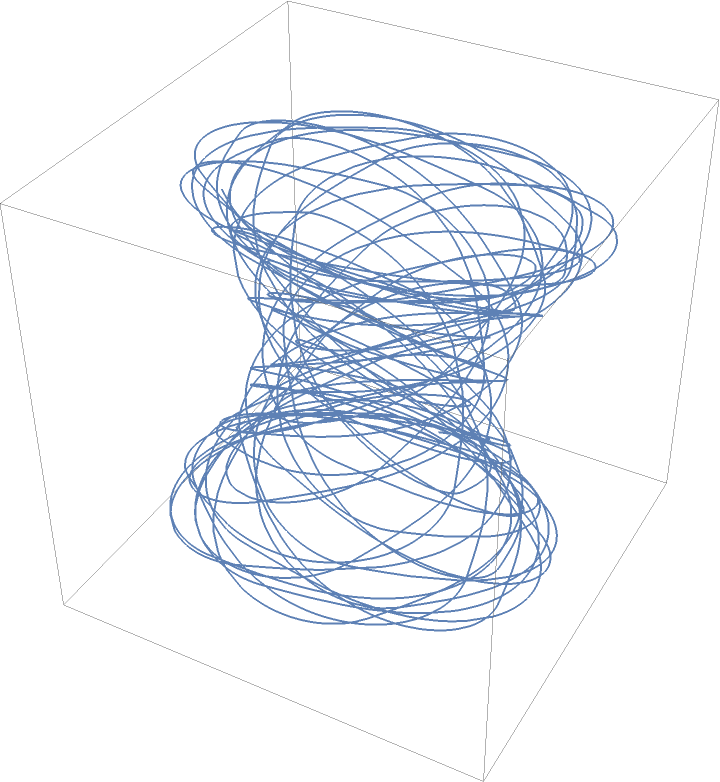Increasing torsion ends in a closed curve:

 In:=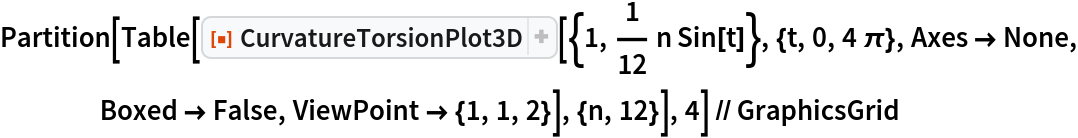Out=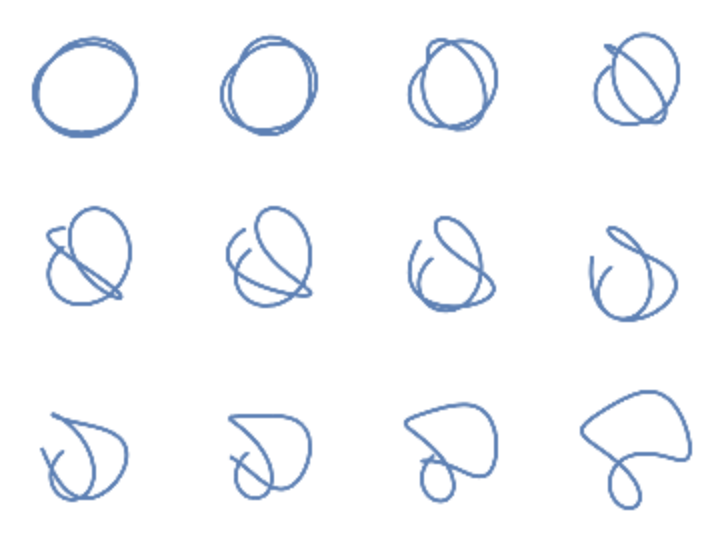All graphics together:

 In:=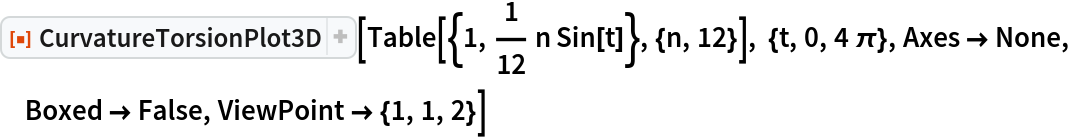Out=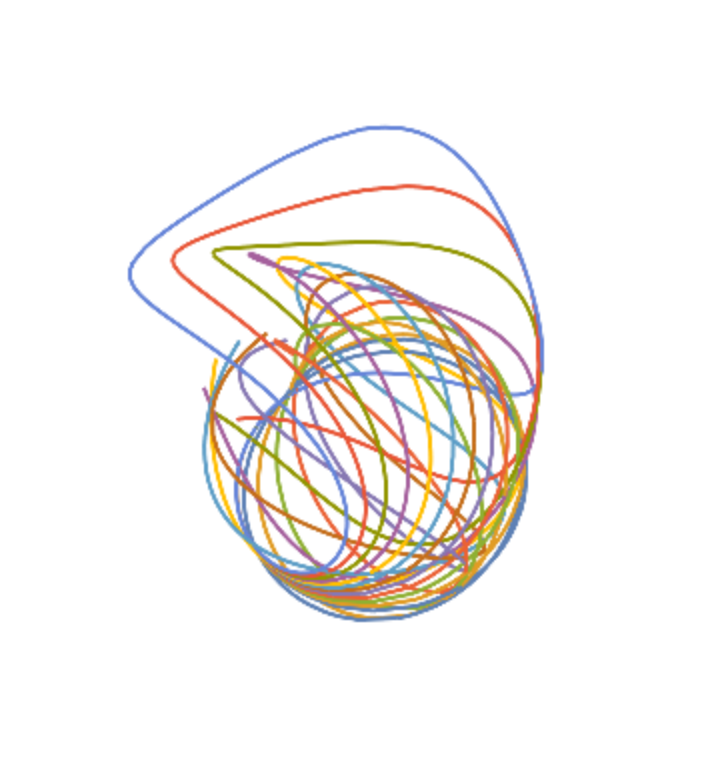Animate the evolution of the curve:

 In:=Out=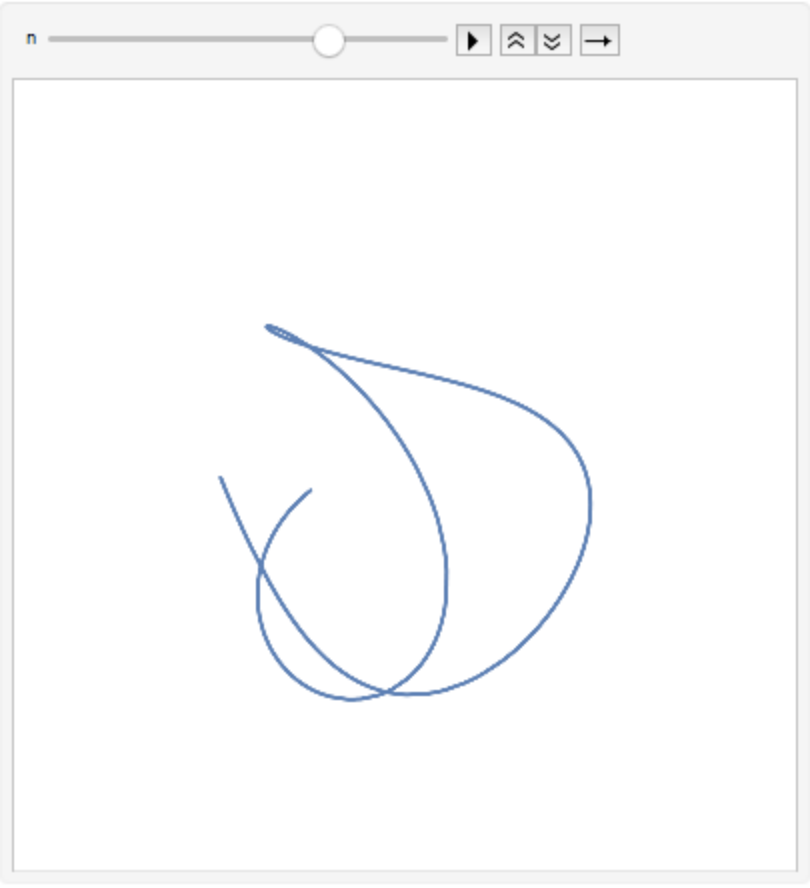### Options

#### ColorFunction

Apply a color function:

 In:=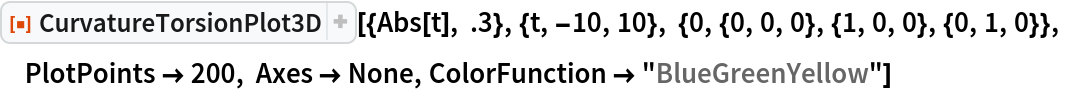Out=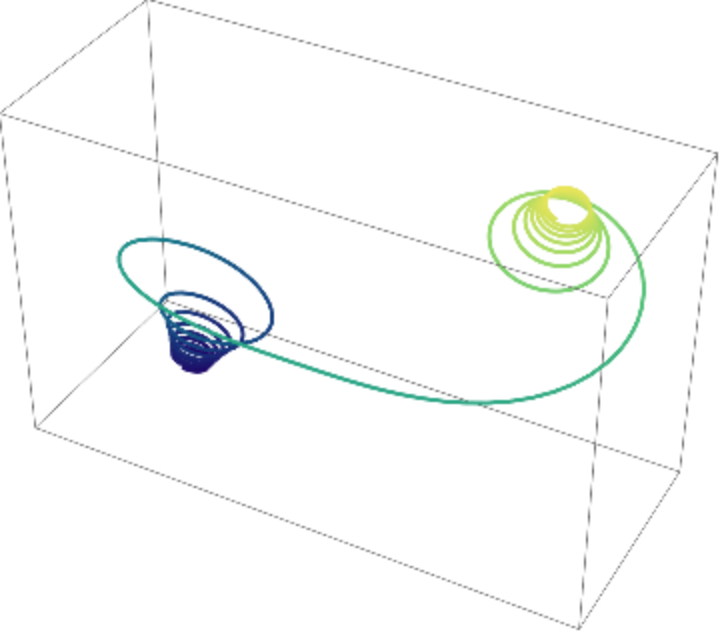### Properties and Relations

Code to get a curve with prescribed curvature (intrinsic curvature):

 In:=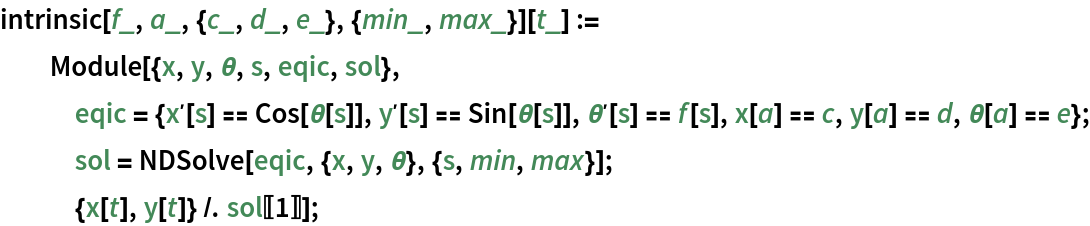Plot a surface of revolution:

 In:=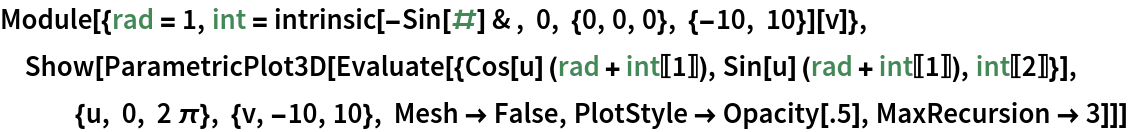Out=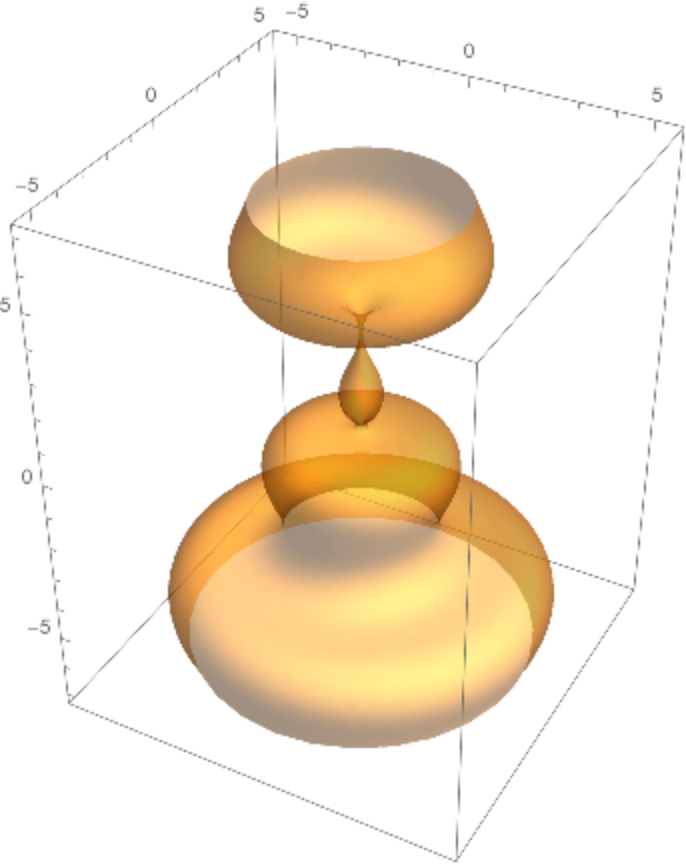Another way to generate a surface with from a curve with intrinsic curvature is by using GeneralizedHelicoid:

 In:=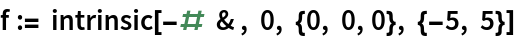In:=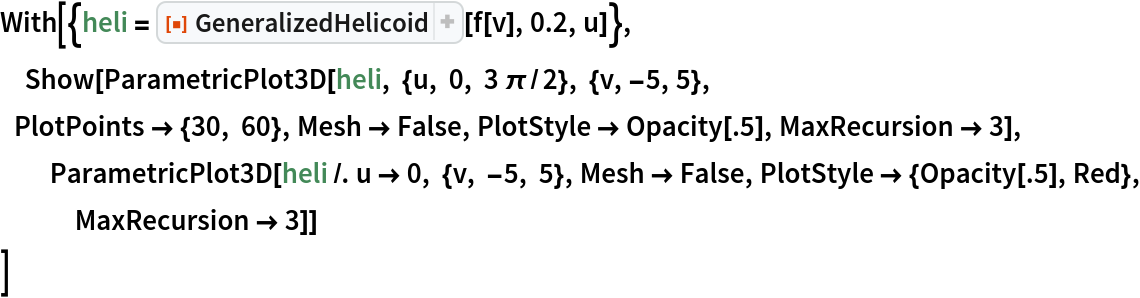Out=